# DataTypes in MongoDB

• Difficulty Level : Expert
• Last Updated : 09 Jun, 2022

In MongoDB, the documents are stores in BSON, which is the binary encoded format of JSON and using BSON we can make remote procedure calls in MongoDB. BSON data format supports various data-types. Below are the enlisted MongoDB data types:

1. String: This is the most commonly used data type in MongoDB to store data, BSON strings are of UTF-8. So, the drivers for each programming language convert from the string format of the language to UTF-8 while serializing and de-serializing BSON. The string must be a valid UTF-8.

Example: In the following example we are storing the name of the student in the student collection: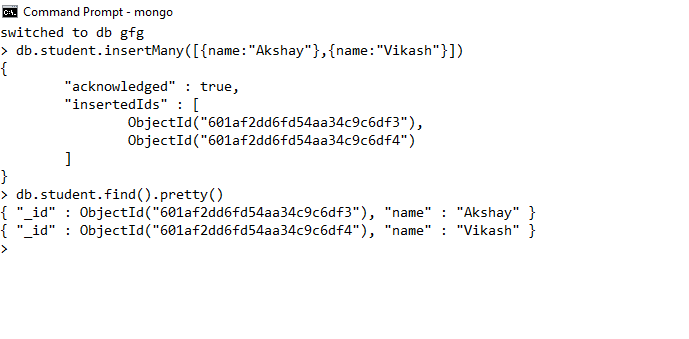Here, the data type of the value of the name field is a string.

2. Integer: In MongoDB, the integer data type is used to store an integer value. We can store integer data type in two forms 32 -bit signed integer and 64 – bit signed integer.

Example: In the following example we are storing the age of the student in the student collection: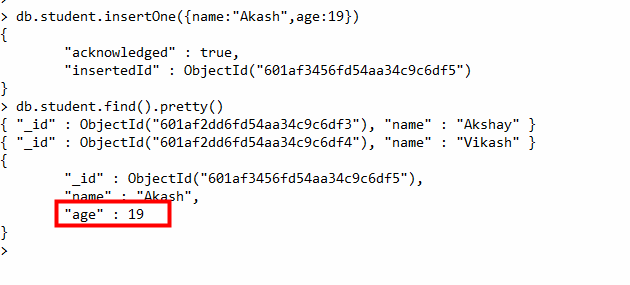3. Double: The double data type is used to store the floating-point values.

Example: In the following example we are storing the marks of the student in the student collection: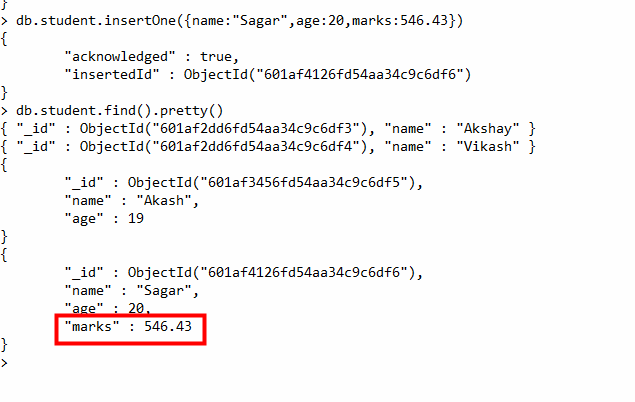4. Boolean: The boolean data type is used to store either true or false.

Example: In the following example we are storing the final result of the student as pass or fail in boolean values.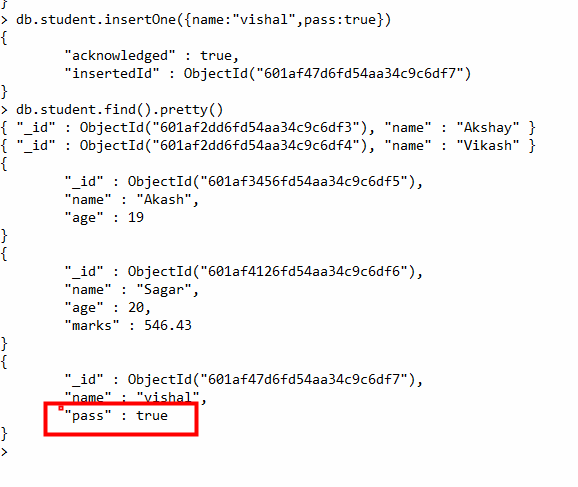5. Null: The null data type is used to store the null value.

Example: In the following example,  the student does not have a mobile number so the number field contains the value null.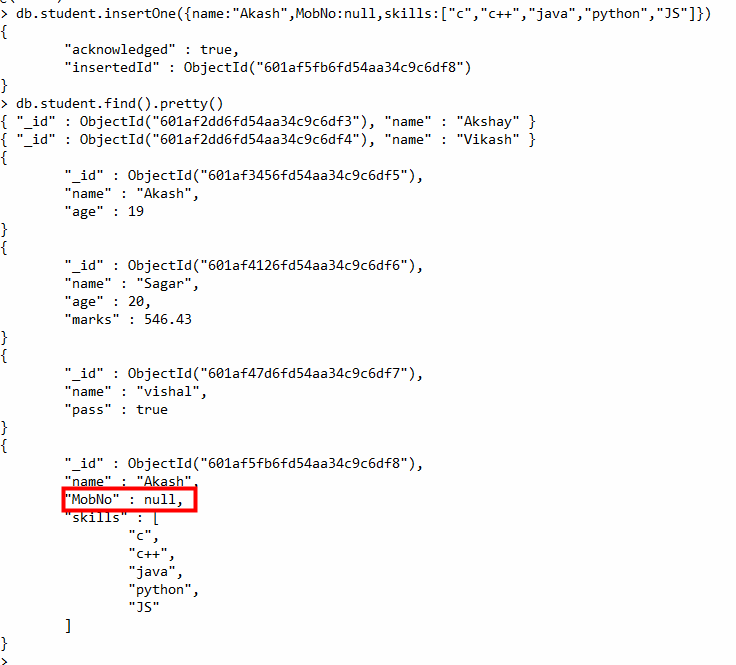6. Array: The Array is the set of values. It can store the same or different data types values in it. In MongoDB, the array is created using square brackets([]).

Example: In the following example, we are storing the technical skills of the student as an array.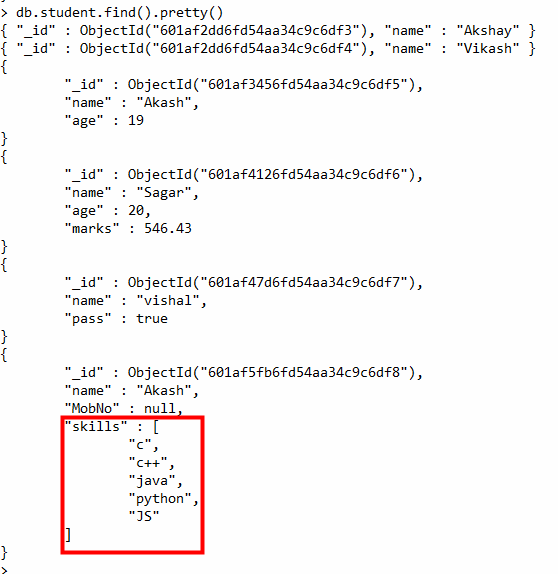7. Object: Object data type stores embedded documents. Embedded documents are also known as nested documents. Embedded document or nested documents are those types of documents which contain a document inside another document.

Example: In the following example, we are storing all the information about a book in an embedded document.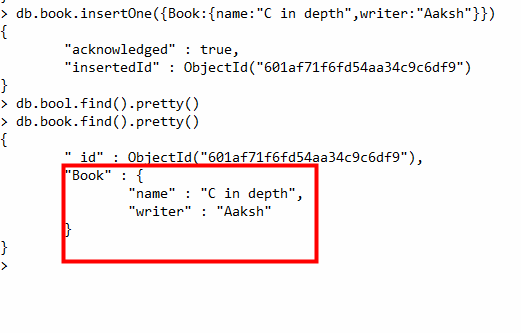8. Object Id: Whenever we create a new document in the collection MongoDB automatically creates a unique object id for that document(if the document does not have it). There is an _id field in MongoDB for each document. The data which is stored in Id is of hexadecimal format and the length of the id is 12 bytes which consist:

• 4-bytes for Timestamp value.
• 5-bytes for Random values. i.e., 3-bytes for machine Id and 2-bytes for process Id.
• 3- bytes for Counter

You can also create your own id field, but make sure that the value of that id field must be unique.

Example: In the following example, when we insert a new document it creates a new unique object id for it.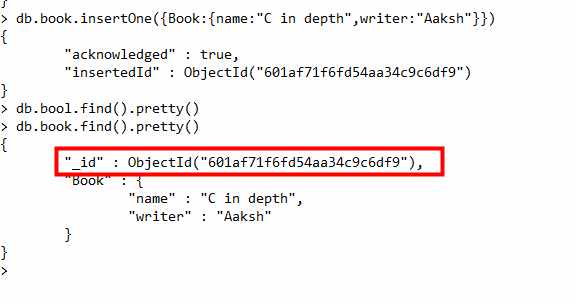9. Undefined: This data type stores the undefined values.

Example: In the following example the type of the duration of the project is undefined.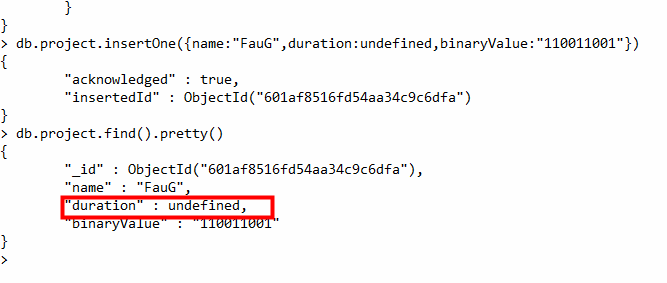10. Binary Data: This datatype is used to store binary data.

Example: In the following example the value stored in the binaryValue field is of binary type.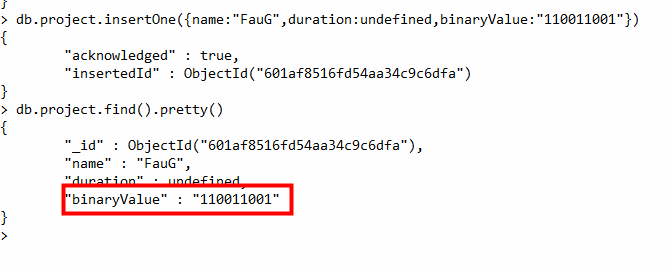11. Date: Date data type stores date. It is a 64-bit integer which represents the number of milliseconds. BSON data type generally supports UTC datetime and it is signed. If the value of the date data type is negative then it represents the dates before 1970. There are various methods to return date, it can be returned either as a string or as a date object. Some method for the date:

• Date(): It returns the current date in string format.
• new Date(): Returns a date object. Uses the ISODate() wrapper.
• new ISODate(): It also returns a date object. Uses the ISODate() wrapper.

Example: In the following example we are using all the above method of the date: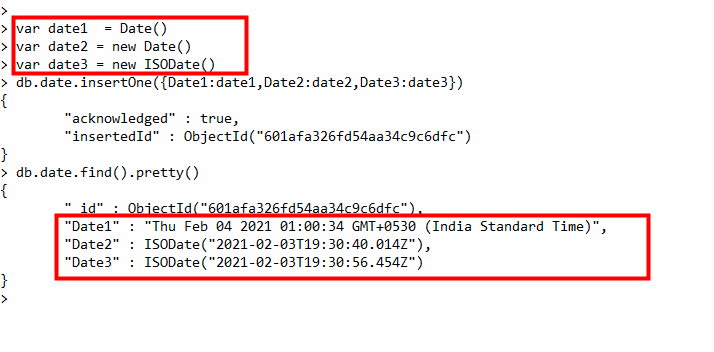12. Min & Max key: Min key compares the value of the lowest BSON element and Max key compares the value against the highest BSON element. Both are internal data types.

Example: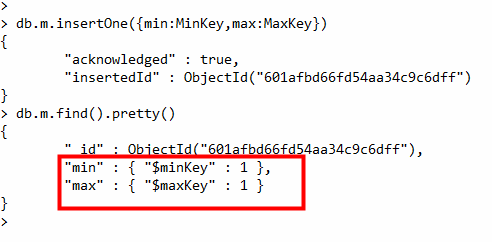13. Symbol: This data type similar to the string data type. It is generally not supported by a mongo shell, but if the shell gets a symbol from the database, then it converts this type into a string type.

Example: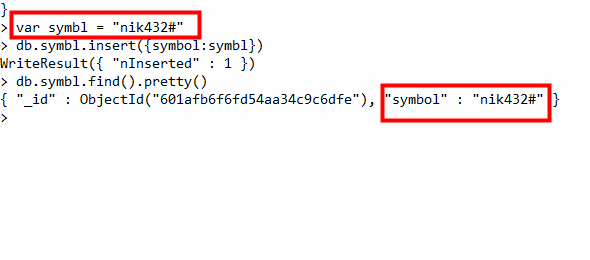14. Regular Expression: This datatype is used to store regular expressions.

Example: In the following example we are storing the regular expression gfg: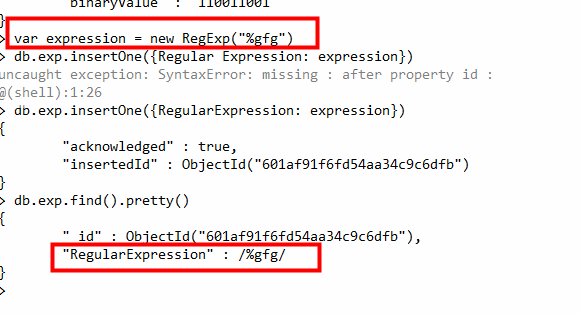15. JavaScript: This datatype is used to store JavaScript code into the document without the scope.

Example: In this example, we are using the JavaScript syntax in the shell:16. JavaScript with Scope: This MongoDB data type store JavaScript data with a scope. This data type is deprecated in MongoDB 4.4.

Example: In this example, we are using the JavaScript syntax in the shell:17. Timestamp: In MongoDB, this data type is used to store a timestamp. It is useful when we modify our data to keep a record and the value of this data type is 64-bit. The value of the timestamp data type is always unique.

Example: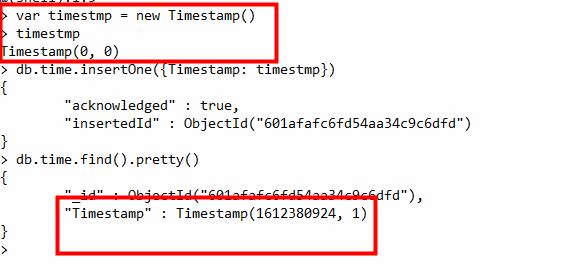18. Decimal: This MongoDB data type store 128-bit decimal-based floating-point value. This data type was introduced in MongoDB version 3.4

Example: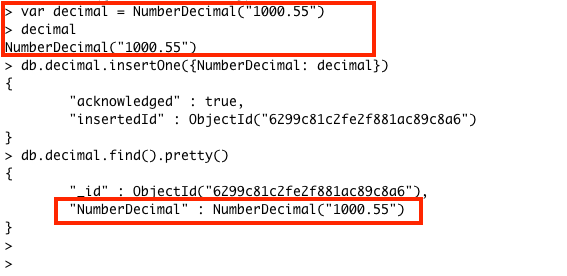My Personal Notes arrow_drop_up